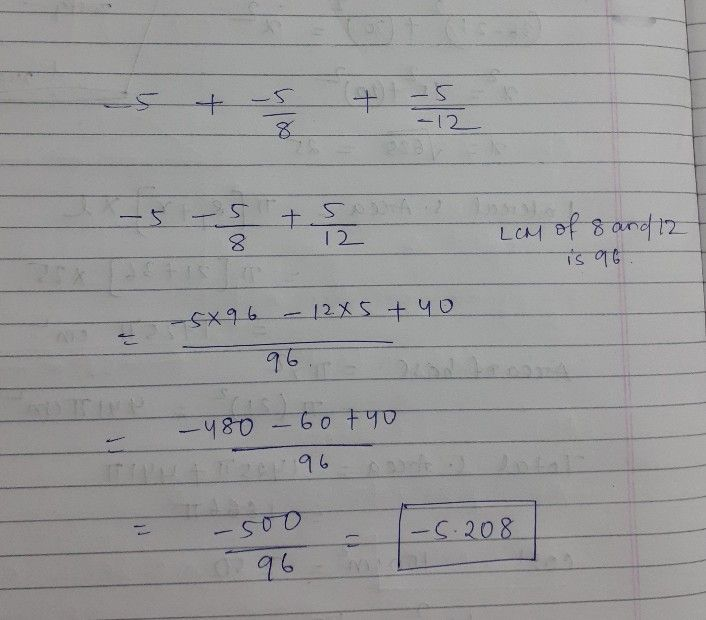Symbol
Problem$lnln0a$ $°-$ $-5+5$ $+$ $=5$ $-8$ $-12$
7th-9th grade
Other
SolutionQanda teacher - saishreeStudent
Thank you